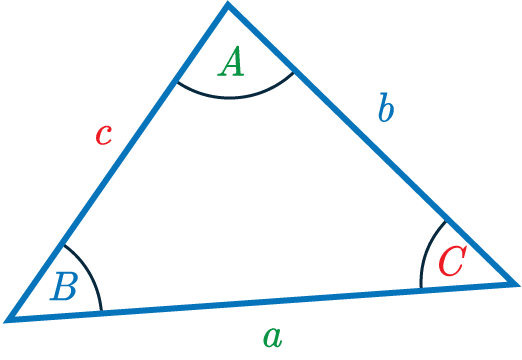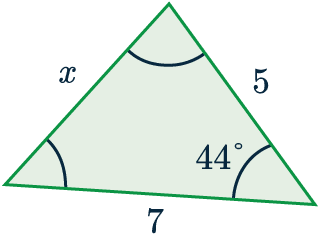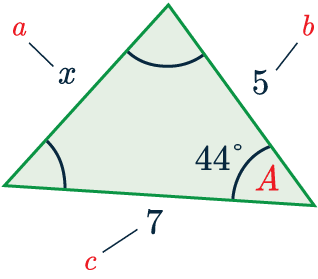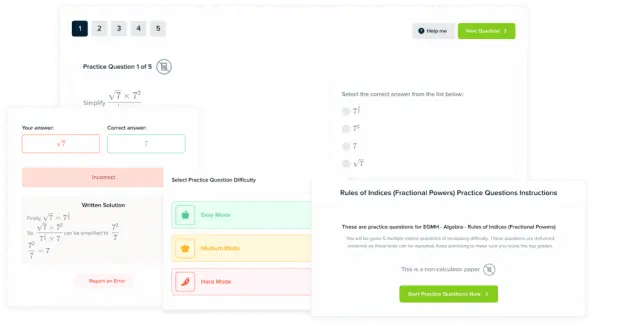# Cosine Rule

GCSELevel 6-7AQACambridge iGCSEEdexcelEdexcel iGCSEOCRWJEC

## Cosine Rule

When we first learn the cosine function, we learn how to use it to find missing side-lengths & angles in right-angled triangles. The cosine rule is an equation that can help us find missing side-lengths and angles in any triangle.

Make sure you are happy with the following topics before continuing:

Level 6-7GCSEAQAEdexcelOCRWJECCambridge iGCSEEdexcel iGCSE

## Cosine Rule Formula

The cosine rule is an equation that helps us find missing side-lengths and angles in any triangle.

It is expressed according to the triangle on the right.

The cosine rule is

$\textcolor{limegreen}{a}^2=\textcolor{blue}{b}^2+\textcolor{red}{c}^2-2\textcolor{blue}{b}\textcolor{red}{c}\cos \textcolor{limegreen}{A}$

In this topic, we’ll go through examples of cosine rule questions.Level 6-7GCSEAQAEdexcelOCRWJECCambridge iGCSEEdexcel iGCSE

## Example: Cosine rule to find a length

Use the cosine rule to find the side-length marked $x$ to $1$ dp.

[3 marks]First we match up the information in the question to the letters in the formula.

$a=x$, $A = 44\degree$$b=5$ and $c=7$.So, we’re ready to substitute the values into the formula.

$x^2=5^2+7^2-(2\times5\times7\times\cos(44))=25+49-70\cos(44)$

Taking the square root of both sides, and putting it into the calculator, we get

$x=\sqrt{25+49-70\cos(44)}=4.9$ ($1$ dp).

Level 6-7GCSEAQAEdexcelOCRWJECCambridge iGCSEEdexcel iGCSE

## Cosine Rule Example Questions

Firstly, we need appropriately label the sides of this triangle. Firstly, we set $a=x$, and therefore we get that $A=19$, since it is the angle opposite. It doesn’t matter how we label the other two sides, so here we’ll let $b=86$ cm and $c=65$ cm. Now, subbing these values into the cosine rule equation, we get

$x^2=86^2+65^2-(2\times86\times65\times\cos(19\degree))=7,396+4,225-11,180\cos(19\degree)$

Then, taking the square root, and putting it into the calculator, we get,

$x=\sqrt{7,396+4,225-11,180\cos(19\degree)}=32$ cm ($2$ sf).Gold Standard Education

As always, we must label our triangle. Firstly, assign the thing we’re looking for to be $A=x$, and therefore make the side opposite to it $a=6$ mm. Then, it doesn’t matter how we choose the other two sides, so we will let $b=5$ mm and $c=7$ mm.

Here, we will use the rearranged version of the formula that looks like

$\cos A=\dfrac{b^2+c^2-a^2}{2bc},$

So, subbing these values into the equation, we get

\begin{aligned} \cos x &=\dfrac{5^2+7^2-6^2}{2\times5\times7} \\ \\ &=\dfrac{25+49-36}{70}\end{aligned}

Taking $\cos^{-1}$ of both sides, and putting it into a calculator, we get

$x=\cos^{-1}\left(\dfrac{25+49-36}{70}\right)=57.1\degree$ ($3$ sf).Gold Standard Education

Firstly, we need appropriately label the sides of this triangle. Firstly, we set $a=x$, and therefore we get that $A=40\degree$, since it is the angle opposite. It doesn’t matter how we label the other two sides, so here we’ll let $b=6$ cm and $c=4.5$ cm. Now, substiuting  these values into the cosine rule equation, we get,

$x^2=6^2+4.5^2-(2\times6\times4.5\times\cos(40\degree))=36+20.25-54\cos(40\degree)$

Then, taking the square root, and putting it into the calculator, we get,

$x=\sqrt{56.25-54\cos(40\degree)}=3.9$ cm ($2$ sf).Gold Standard Education

We can use the rearranged version of the formula that looks like,

$\cos A=\dfrac{b^2+c^2-a^2}{2bc}$

So, subbing these values into the equation, we get

\begin{aligned} \cos x &=\dfrac{7^2+10^2-12^2}{2\times7\times10} \\ \\ &=\dfrac{49+100-144}{140} \end{aligned}

Taking $\cos^{-1}$ of both sides, and putting it into a calculator, we get

$x=\cos^{-1}\left(\dfrac{5}{140}\right)=88.0\degree$ ($1$ dp).Gold Standard Education

Substituting values into the cosine rule equation, we get,

$x^2=5.2^2+3.5^2-(2\times5.2\times3.5\times\cos(28\degree))=27.04+12.25-36.4\cos(28\degree)$

Then, taking the square root, and putting it into the calculator, we get,

$x=\sqrt{39.29-36.4\cos(28\degree)}=2.7$ cm ($2$ sf).Gold Standard Education

## Cosine Rule Worksheet and Example Questions

### (NEW) Cosine Rule Exam Style Questions -MME

Level 6-7GCSENewOfficial MME

### (NEW) Sine and Cosine rule mixed Exam Style Questions -MME

Level 6-7Level 8-9GCSENewOfficial MME

## Cosine Rule Drill Questions

### Cosine Rule - Drill Questions

Level 6-7GCSEProduct

### £19.99

/month

Learn an entire GCSE course for maths, English and science on the most comprehensive online learning platform. With revision explainer videos & notes, practice questions, topic tests and full mock exams for each topic on every course, it’s easy to Learn and Revise with the MME Learning Portal.

Level 4-5GCSE

Level 6-7GCSE